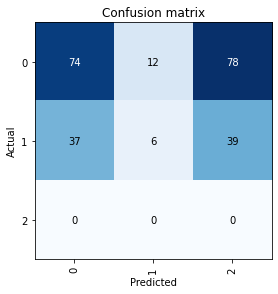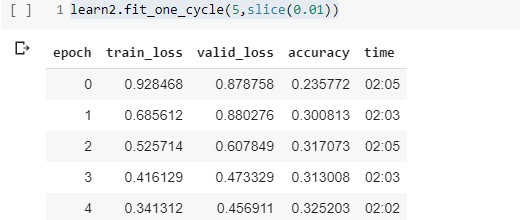# Serious error in confusion matrix of fastaiIn confusion matrix it is showing that there is no Class 2 data and so there is no prediction.
My question is how it can be possible because when i look on my data i found the images of class 2 and even when i do : learn2.show_results(rows=3, figsize=(12,15))
then also

Is there anybody who can deal with my problem??

Hi Akshat!

Can you write how are you defining the learner? And how are you plotting the confusion matrix?

1 Like

np.random.seed(42)

src = (ImageList.from_csv(path, ‘a. IDRiD_Disease Grading_Training Labels.csv’, folder=‘a. Training Set’, suffix=’.jpg’).split_by_rand_pct(0.2).label_from_df(label_delim=’ '))

data = (src.transform(size=128).databunch().normalize(imagenet_stats))

data.show_batch(rows=3)

learn2 = cnn_learner(data,models.resnet50,metrics = accuracy)

from torchsummary import summary
summary(learn2.model,input_size=(3, 128, 128))

learn2.fit_one_cycle(5,slice(0.01))interp = ClassificationInterpretation.from_learner(learn2)
interp.plot_confusion_matrix()We can see the ground truth and prediction images where you will find that all the class of image are present i.e. Class 1 , Class 2, Class 3.
But in confusing matrix it is not showing actual image of Class 3 in the matrix.
I don’t know why it is happening?
And note that data.c is also giving 3 as output.

As I can see in the attached image, your classifier is multi-label, right?
If so, I think you can’t use the `plot_confusion_matrix()` function of the `ClassificationInterpretation`. You may have to define another function for multi-label.

can you suggest any function??

I can tell you some websites that may be useful to you, but you may have to create your own function:

1. In fastai2 there is a class called `Interpretation()` which is useful for interpreting multi-label classifiers. Even though it doesn’t have the `confusion_matrix()` function, it does have others that may help you, like `plot_top_losses()`.

2. Sklearn has a metric called `multilabel_confusion_matrix()` you can check it out too.

I hope some of this will help youI am still confused as Jeremy Howard in its second lesson show the model which do teddy bear classification

One more thing is that my dataset is multiclass not multilabel.

And if my model is doing multilabel classification instead of multiclass then do you know how can we train our model in fastai in such a way that it performs only multiclass not multilabel.

@Saioa Thank you for your kind help!
I detected some problems and change some initial codes when I was trying to read my data from CSV file and now it is working and the confusion matrix is also showing the results.
Thanks.

2 Likes